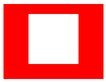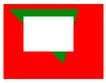# How to clear the canvas using clearRect in HTML ?

The clearRect() method of the Canvas 2D API which is used to erase the pixel in a rectangular area by setting the pixel color to transparent black (rgba(0, 0, 0, 0)).

Syntax:

`abc.clearRect(x, y, width, height);`

Paramaters:

• x, y: These paramter represents the top-left coordinate of the rectangular box.
• width: It is used to set the width of the rectangular box
• height: It is used to set the of the rectangular box

Example 1: In the following examplen the `clearRect()` method is used to clear the pixel of a rectangle of size (200×300) from (50, 20).

 ` ` `<``html` `lang``=``"en"``> ` ` `  `<``head``> ` `    ``<``title``> ` `        ``How to clear the canvas ` `        ``using clearRect in HTML? ` `    `` ` ` ` ` `  `<``body``> ` `    ``<``canvas` `id``=``"canvas"``> ` ` `  `    ``<``script``> ` `        ``const canvas = document.getElementById("canvas"); ` `        ``const abc = canvas.getContext('2d'); ` `     `  `        ``abc.beginPath(); ` `        ``abc.fillStyle = "red"; ` `        ``abc.fillRect(0, 0, 200, 300); ` `     `  `        ``abc.clearRect(50, 20, 100, 100); ` `    `` ` ` ` ` `  ` `

Output:Example 2:

 ` ` `<``html` `lang``=``"en"``> ` ` `  `<``head``> ` `    ``<``title``> ` `        ``How to clear the canvas ` `        ``using clearRect in HTML? ` `    `` ` ` ` ` `  `<``body``> ` `    ``<``canvas` `id``=``"canvas"``> ` ` `  `    ``<``script``> ` `        ``const canvas = document.getElementById("canvas"); ` `        ``const abc = canvas.getContext('2d'); ` `          `  `        ``// Draws a rectangle of 200x300 ` `        ``abc.beginPath(); ` `        ``abc.fillStyle = "red"; ` `        ``abc.fillRect(0, 0, 200, 300); ` `          `  `        ``// Draws a traiangle ` `        ``abc.beginPath(); ` `        ``abc.fillStyle = "green"; ` `        ``abc.moveTo(10, 10); ` `        ``abc.lineTo(150, 10); ` `        ``abc.lineTo(120, 120); ` `        ``abc.closePath(); ` `        ``abc.fill(); ` `      `  `        ``abc.clearRect(40, 25, 100, 70); ` `    `` ` ` ` ` `  ` `

Output:Example 3: In this example we will erase whole canvas.

 ` ` `<``html` `lang``=``"en"``> ` ` `  `<``head``> ` `    ``<``title``> ` `        ``How to clear the canvas ` `        ``using clearRect in HTML? ` `    `` ` ` ` ` `  `<``body``> ` `    ``<``canvas` `id``=``"canvas"``> ` ` `  `    ``<``script``> ` `        ``const canvas = document.getElementById("canvas"); ` `        ``const abc = canvas.getContext('2d'); ` `      `  `        ``abc.beginPath(); ` `        ``abc.fillStyle = "red"; ` `        ``abc.fillRect(0, 0, 200, 300); ` `          `  `        ``// This line will erase whole canvas and ` `        ``// we will get an empty screen ` `        ``abc.clearRect(0, 0, canvas.width, canvas.height); ` `    `` ` ` ` ` `  ` `

Note: Be aware that `clearRect()` may cause unintended side effects if you’re not using paths properly. Make sure to call beginPath() before starting to draw new items after calling `clearRect()`.My Personal Notes arrow_drop_upCheck out this Author's contributed articles.

If you like GeeksforGeeks and would like to contribute, you can also write an article using contribute.geeksforgeeks.org or mail your article to contribute@geeksforgeeks.org. See your article appearing on the GeeksforGeeks main page and help other Geeks.

Please Improve this article if you find anything incorrect by clicking on the "Improve Article" button below.

Article Tags :
Practice Tags :

Be the First to upvote.

Please write to us at contribute@geeksforgeeks.org to report any issue with the above content.